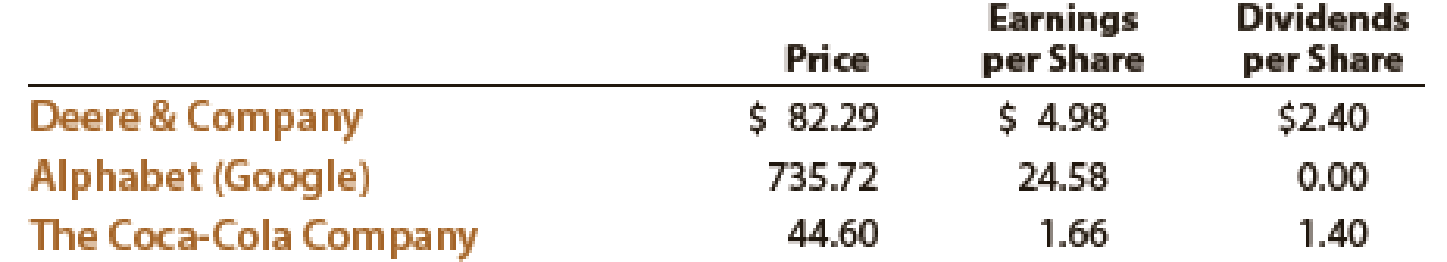Chapter 17, Problem 22E

Chapter
Section
Textbook Problem
1 views

# Price-earnings ratio; dividend yieldThe table that follows shows the stock price, earnings per share, and dividends per share for three companies for a recent year:a. Determine the price-earnings ratio and dividend yield for the three companies. Round ratios and percentages to one decimal place as appropriate. b. Explain the differences in these ratios across the three companies.

(a) (1)

To determine

Determine Price earnings ratio.

Explanation

Financial Ratios: Financial ratios are the metrics used to evaluate the liquidity, capabilities, profitability, and overall performance of a company.

Price/earnings ratio is used to determine the profitability of a company. This ratio is abbreviated as P/E.

Formula:

Price/earnings ratio= Market price per share of common stockEarnings per share

Calculate Price earnings ratio for D&C.

Price/Earnings ratio = Market price per shareEarnings per share=$82.29per share$4.98per share=16.5

Calculate Price earnings ratio for A

(2)

To determine

Determine Dividend yield.

(b)

To determine

Explain the differences in the ratio across the companies.

### Still sussing out bartleby?

Check out a sample textbook solution.

See a sample solution

#### The Solution to Your Study Problems

Bartleby provides explanations to thousands of textbook problems written by our experts, many with advanced degrees!

Get Started

#### Find more solutions based on key concepts